# mne.minimum_norm.apply_inverse#

mne.minimum_norm.apply_inverse(evoked, inverse_operator, lambda2=0.1111111111111111, method='dSPM', pick_ori=None, prepared=False, label=None, method_params=None, return_residual=False, use_cps=True, verbose=None)[source]#

Apply inverse operator to evoked data.

Parameters:
evoked`Evoked` object

Evoked data.

inverse_operatorinstance of `InverseOperator`

Inverse operator.

lambda2`float`

The regularization parameter.

method“MNE” | “dSPM” | “sLORETA” | “eLORETA”

Use minimum norm , dSPM (default) , sLORETA , or eLORETA .

pick_ori`None` | “normal” | “vector”

Options:

• `None`

Pooling is performed by taking the norm of loose/free orientations. In case of a fixed source space no norm is computed leading to signed source activity.

• `"normal"`

Only the normal to the cortical surface is kept. This is only implemented when working with loose orientations.

• `"vector"`

No pooling of the orientations is done, and the vector result will be returned in the form of a `mne.VectorSourceEstimate` object.

preparedbool

If True, do not call `prepare_inverse_operator()`.

label

Restricts the source estimates to a given label. If None, source estimates will be computed for the entire source space.

method_params

Additional options for eLORETA. See Notes for details.

New in version 0.16.

return_residualbool

If True (default False), return the residual evoked data. Cannot be used with `method=='eLORETA'`.

New in version 0.17.

use_cpsbool

Whether to use cortical patch statistics to define normal orientations for surfaces (default True).

Only used when the inverse is free orientation (`loose=1.`), not in surface orientation, and `pick_ori='normal'`.

New in version 0.20.

verbose

Control verbosity of the logging output. If `None`, use the default verbosity level. See the logging documentation and `mne.verbose()` for details. Should only be passed as a keyword argument.

Returns:
stc

The source estimates.

residualinstance of `Evoked`

The residual evoked data, only returned if return_residual is True.

`apply_inverse_raw`

Apply inverse operator to raw object.

`apply_inverse_epochs`

Apply inverse operator to epochs object.

Notes

Currently only the `method='eLORETA'` has additional options. It performs an iterative fit with a convergence criterion, so you can pass a `method_params` `dict` with string keys mapping to values for:

‘eps’float

The convergence epsilon (default 1e-6).

‘max_iter’int

The maximum number of iterations (default 20). If less regularization is applied, more iterations may be necessary.

‘force_equal’bool

Force all eLORETA weights for each direction for a given location equal. The default is None, which means `True` for loose-orientation inverses and `False` for free- and fixed-orientation inverses. See below.

The eLORETA paper  defines how to compute inverses for fixed- and free-orientation inverses. In the free orientation case, the X/Y/Z orientation triplet for each location is effectively multiplied by a 3x3 weight matrix. This is the behavior obtained with `force_equal=False` parameter.

However, other noise normalization methods (dSPM, sLORETA) multiply all orientations for a given location by a single value. Using `force_equal=True` mimics this behavior by modifying the iterative algorithm to choose uniform weights (equivalent to a 3x3 diagonal matrix with equal entries).

It is necessary to use `force_equal=True` with loose orientation inverses (e.g., `loose=0.2`), otherwise the solution resembles a free-orientation inverse (`loose=1.0`). It is thus recommended to use `force_equal=True` for loose orientation and `force_equal=False` for free orientation inverses. This is the behavior used when the parameter `force_equal=None` (default behavior).

References

## Examples using `mne.minimum_norm.apply_inverse`#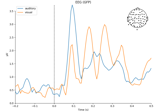Overview of MEG/EEG analysis with MNE-Python

Overview of MEG/EEG analysis with MNE-Python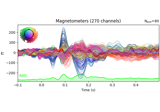Working with CTF data: the Brainstorm auditory dataset

Working with CTF data: the Brainstorm auditory dataset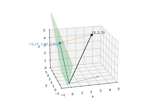Background on projectors and projections

Background on projectors and projections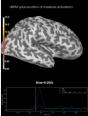Source localization with MNE, dSPM, sLORETA, and eLORETA

Source localization with MNE, dSPM, sLORETA, and eLORETA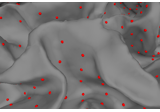The role of dipole orientations in distributed source localization

The role of dipole orientations in distributed source localization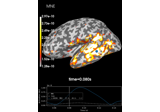Computing various MNE solutions

Computing various MNE solutions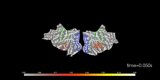Visualize source time courses (stcs)

Visualize source time courses (stcs)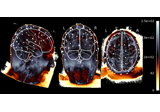EEG source localization given electrode locations on an MRI

EEG source localization given electrode locations on an MRI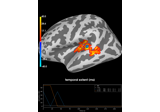Permutation t-test on source data with spatio-temporal clustering

Permutation t-test on source data with spatio-temporal clustering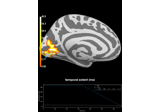Repeated measures ANOVA on source data with spatio-temporal clustering

Repeated measures ANOVA on source data with spatio-temporal clustering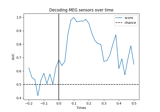Decoding (MVPA)

Decoding (MVPA)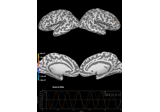Corrupt known signal with point spread

Corrupt known signal with point spread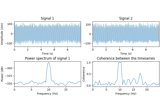DICS for power mapping

DICS for power mapping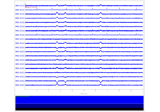Simulate raw data using subject anatomy

Simulate raw data using subject anatomy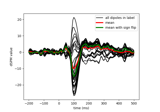Compute MNE-dSPM inverse solution on single epochs

Compute MNE-dSPM inverse solution on single epochs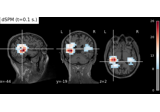Compute MNE-dSPM inverse solution on evoked data in volume source space

Compute MNE-dSPM inverse solution on evoked data in volume source space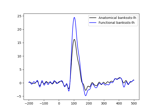Generate a functional label from source estimates

Generate a functional label from source estimates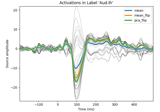Extracting the time series of activations in a label

Extracting the time series of activations in a label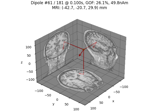Compute sparse inverse solution with mixed norm: MxNE and irMxNE

Compute sparse inverse solution with mixed norm: MxNE and irMxNE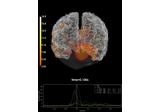Compute MNE inverse solution on evoked data with a mixed source space

Compute MNE inverse solution on evoked data with a mixed source space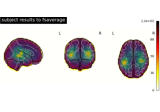Morph volumetric source estimate

Morph volumetric source estimate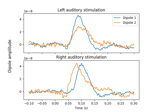Computing source timecourses with an XFit-like multi-dipole model

Computing source timecourses with an XFit-like multi-dipole model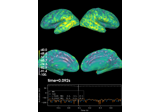Computing source space SNR

Computing source space SNR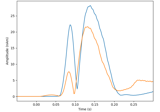Compute MxNE with time-frequency sparse prior

Compute MxNE with time-frequency sparse prior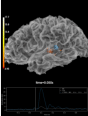Plotting the full vector-valued MNE solution

Plotting the full vector-valued MNE solution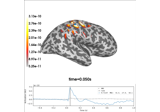Optically pumped magnetometer (OPM) data

Optically pumped magnetometer (OPM) dataFrom raw data to dSPM on SPM Faces dataset

From raw data to dSPM on SPM Faces dataset